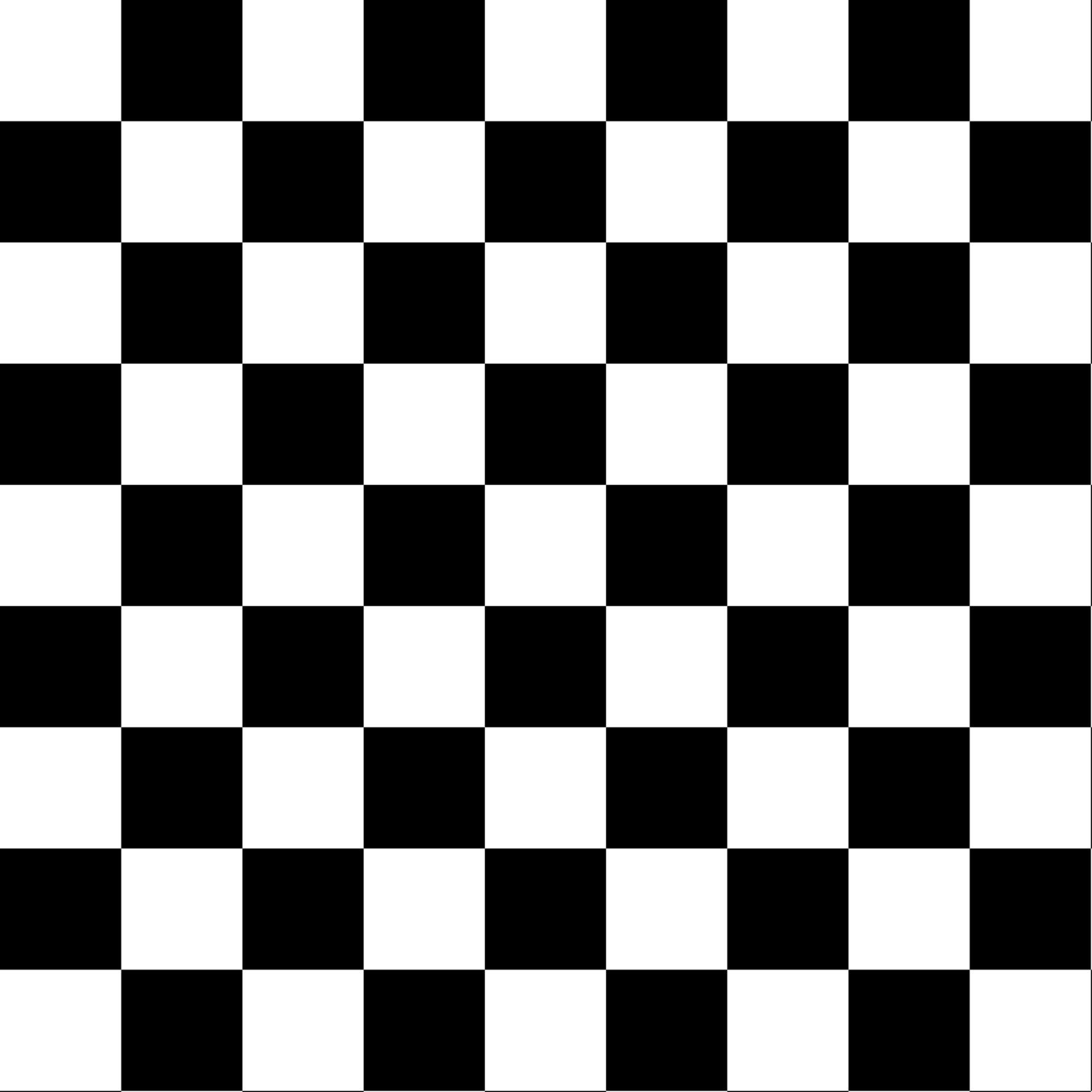This generator supplies 0 or 1 value depending on the provided coordinates position on a checkerboard. The frequency determines the number of squares per unit.

gen_checkerboard(x, y = NULL, z = NULL, t = NULL, frequency = 1, ...)

## Arguments

x, y, z, t The coordinates to get pattern from The frequency of the generator ignored

## Value

A numeric vector

Other Pattern generators: gen_spheres(), gen_waves()
grid <- long_grid(seq(1, 10, length.out = 1000), seq(1, 10, length.out = 1000))
grid$chess <- gen_checkerboard(grid$x, grid\$y)